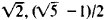# Algebraic Number

(redirected from Algebraics)
Also found in: Dictionary, Thesaurus.

## number

number, entity describing the magnitude or position of a mathematical object or extensions of these concepts.

### The Natural Numbers

Cardinal numbers describe the size of a collection of objects; two such collections have the same (cardinal) number of objects if their members can be matched in a one-to-one correspondence. Ordinal numbers refer to position relative to an ordering, as first, second, third, etc. The finite cardinal and ordinal numbers are called the natural numbers and are represented by the symbols 1, 2, 3, 4, etc. Both types can be generalized to infinite collections, but in this case an essential distinction occurs that requires a different notation for the two types (see transfinite number).

### The Integers and Rational Numbers

To the natural numbers one adjoins their negatives and zero to form the integers. The ratios a/b of the integers, where a and b are integers and b ≠ 0, constitute the rational numbers; the integers are those rational numbers for which b = 1. The rational numbers may also be represented by repeating decimals; e.g., 1/2 = 0.5000 … , 2/3 = 0.6666 … , 2/7 = 0.285714285714 … (see decimal system).

### The Real Numbers

The real numbers are those representable by an infinite decimal expansion, which may be repeating or nonrepeating; they are in a one-to-one correspondence with the points on a straight line and are sometimes referred to as the continuum. Real numbers that have a nonrepeating decimal expansion are called irrational, i.e., they cannot be represented by any ratio of integers. The Greeks knew of the existence of irrational numbers through geometry; e.g., √2 is the length of the diagonal of a unit square. The proof that √2 is unable to be represented by such a ratio was the first proof of the existence of irrational numbers, and it caused tremendous upheaval in the mathematical thinking of that time.

### The Complex Numbers

Numbers of the form z = x + yi, where x and y are real and i = √−1, such as 8 + 7i (or 8 + 7√−1), are called complex numbers; x is called the real part of z and yi the imaginary part. The real numbers are thus complex numbers with y = 0; e.g., the real number 4 can be expressed as the complex number 4 + 0i. The complex numbers are in a one-to-one correspondence with the points of a plane, with one axis defining the real parts of the numbers and one axis defining the imaginary parts. Mathematicians have extended this concept even further, as in quaternions.

### The Algebraic and Transcendental Numbers

A real or complex number z is called algebraic if it is the root of a polynomial equation zn + an − 1zn − 1 + … + a1z + a0 = 0, where the coefficients a0, a1, … an − 1 are all rational; if z cannot be a root of such an equation, it is said to be transcendental. The number √2 is algebraic because it is a root of the equation z2 + 2 = 0; similarly, i, a root of z2 + 1 = 0, is also algebraic. However, F. Lindemann showed (1882) that π is transcendental, and using this fact he proved the impossibility of “squaring the circle” by straight edge and compass alone (see geometric problems of antiquity). The number e has also been found to be transcendental, although it still remains unknown whether e + π is transcendental.

### Bibliography

See G. Ifrah, The Universal History of Numbers (1999).

The following article is from The Great Soviet Encyclopedia (1979). It might be outdated or ideologically biased.

## Algebraic Number

any number ɑ satisfying the algebraic equation a1 an + . . . + aka a + an+1 = 0, where n ≥ 1 and a1, . . . , an and an+1 are whole (rational) numbers. The number ɑ is called an algebraic integer if a1 = 1. If the polynomial f(x) = a1xn+ . . . + anx + an+1 is not the product of two other polynomials of positive degree with rational coefficients, then the number n is called the degree of the algebraic number a. The simplest algebraic numbers are the roots of the binomial equation xn = a, where α is a rational number. For example, algebraic numbers are the rational numbers and the numbersintegral algebraic numbers are the integers and the numbers. Two important trends in number theory are closely associated with the concept of the algebraic number. The first is the arithmetic of algebraic numbers (algebraic theory of numbers), originated by E. Kummer in the middle of the 19th century, which studies properties of algebraic numbers. Algebraic integers possess a number of properties analogous to those of integral rational numbers; however, the unique factorization theorem has no place in the theory of algebraic integers. To preserve unique factorization, Kummer introduced his so-called ideal numbers. The second trend is the theory of approximation of algebraic numbers, which studies the degree of approximation of these numbers by rational numbers or by algebraic numbers themselves. The first result in this direction was the theorem of J. Liouville, which showed that algebraic numbers are “badly” approximated by rational numbers. More precisely, if α is an algebraic number of degree n, then, for any integral rational values of ρ and q, the inequality ǀa − p/q ǀ > C/qn, holds, where C = C(a) > 0 is a constant independent of ρ and q, from which it follows that it is not difficult to construct an arbitrary number of nonalgebraic numbers—the transcendental numbers.

### REFERENCES

Gekke, E. Lektsii po teorii algebraicheskikh chisel. Moscow-Leningrad, 1940. (Translated from German.)
Gel’fond, A. O. Transtsendentnye i algebraicheskie chista. Moscow, 1952.
Borevich, Z. I., and I. R. Shafarevich. Teoriia chisel. Moscow, 1964.

A. A. KARATSUBA